Courses

# Physics: CBSE Sample Question Paper (2020-21)- 3 Notes | EduRev

## Class 12 : Physics: CBSE Sample Question Paper (2020-21)- 3 Notes | EduRev

The document Physics: CBSE Sample Question Paper (2020-21)- 3 Notes | EduRev is a part of the Class 12 Course Sample Papers for Class 12 Medical and Non-Medical.
All you need of Class 12 at this link: Class 12

Class-XII
Physics Theory
TIME: 3 Hrs.
M.M: 70

General Instructions:

(1) All questions are compulsory. There are 33 questions in all.
(2) This question paper has five sections: Section A, Section B, Section C, Section D and Section E.
(3) Section A contains ten very short answer questions and four assertion reasoning MCQs of 1 mark each, Section B has two case-based questions of 4 marks each, Section C contains nine short answer questions of 2 marks each, Section D contains five short answer questions of 3 marks each and Section E contains three long answer questions of 5 marks each.
(4) There is no overall choice. However, an internal choice is provided. You have to attempt only one of the choices in such questions.

Section A

All questions are compulsory. In case of internal choices, attempt any one of them.
Q.1. In the given circuit, what is the potential difference between the A and B ?  (1 Mark)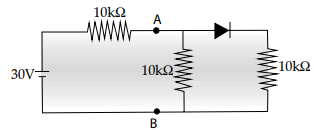Ans: Here diode is forward biased. If diode is ideal, its resistance is zero. The effective
resistance across A and B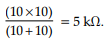Current in the circuit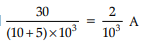So, potential difference across A and B is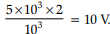Q.2. What is the approximate shape of the equipotential surface at a great distance from a collection of charges whose total sum is not zero?  (1 Mark)
Ans: The equipotential surface, is perpendicular to the field lines. So there must be electric field, which cannot be without charge
So, the algebraic sum of all charges must not be zero. Equipotential surface at a great distance means that space of charge is negligible as compared to distance. So, the collection of charges is considered as a point charge. Electric potential due to point charge is,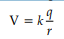which explains that electric potentials due to point charge is same for all equidistant points. The locus of these equidistant points, which are at same potential, forms spherical surface.

OR

How does the angle of minimum deviation of a glass prism vary, if the incident violet light is replaced by red light?   (1 Mark)
Ans: (i) Decreases
(ii) μviolet > μRed
Detailed answer: We know , δm = (n21 – 1) A. Hence, larger the refractive index, larger will be the deviation. As refractive index of glass for violet is more than the refractive index of glass for red, the deviation will decrease if the incident violet light is replaced by red light.

Q.3. A square surface of side L metres is in the plane of the paper. A uniform electric E(volt m–1), also in the plane of the paper, is limited only to the lower half of the square surface as shown in the figure.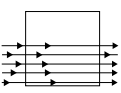What is the electric flux (in SI units) associated with the surface?   (1 Mark)
Ans: As the electric field lines are parallel to the surface of the square, so there will not be any field lines crossing the surface. Hence, the electric flux through the surface will be zero.

Q.4. Why a laser beam is called coherent?  (1 Mark)
Ans: The light coming out of LASER is a monochromatic light i.e. single colored. The single colored light will have the wavelength by which it can be said as coherent as well.

OR

What is the unit of conductance?  (1 Mark)
Ans: The unit of conductance is mho or simen(s).

Q.5. A square of side L meters lies in the x–y plane in a region where the magnetic field is given by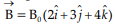Tesla, where B0 is constant. What will be the magnitude of flux passing through the square?  (1 Mark)
Ans: Magnetic flux is defined as the total number of magnetic lines of force passing normally through an area placed in a magnetic field.
Square lies in x-y plane in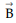.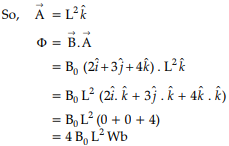Q.6. By using the variations on a Wheatstone bridge, what type of parameters can be measured?  (1 Mark)
Ans: A Wheatstone bridge consists of resistive arms. It is used for the measurement of quantities such as capacitance, inductance and impedance by making use of thevariations because the ratio can be applied to these quantities as well.

Q.7. What do you mean by mass defect of a nucleus? (1 Mark)
Ans: The difference between the rest mass of a nucleus and the sum of the rest masses of its constituent nucleons is called its mass defect. It is given by
∆m=Zmp + (A – Z) mn – M

OR

Name the current that flows in circles inside a disc. (1 Mark)
Ans: The current that flows in circles inside a disc is called Eddy current.

Q.8. What is the approximate wavelength of a photon needed to remove a proton from a nucleus which is bound to the nucleus with 1 MeV energy?  (1 Mark)
Ans: Energy of the photon must be equal to the binding energy of proton So, energy of photon = 1 MeV= 106 × 1.6 × 10–19 J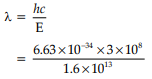= 1.24 × 10–3 × 10–9
= 1.24 × 10–3 nm

OR

If the atomic number of an element is 11 and the atomic mass is 24, how many electrons does it have?  (1 Mark)
Ans: Atomic number of any element gives the number of protons which is equal to the number of electrons in an atom. Sodium has atomic number 11, this shows that Sodium atom has 11 protons in its nucleus and has 11 electrons surrounding its nucleus.

Q.9. A paramagnetic sample shows a net magnetization of 8 Am–1 when placed in an external magnetic field of 0.6 T at a temperature of 4 K. When the same sample is placed in an external magnetic field of 0.2 T at a temperature of 16 K, what will be the magnetization?  (1 Mark)
Ans:  According to the Curie’s law of magnetization, the magnetic susceptibility for a substance is directly proportional to magnetic field induction (B) and inversely proportional to the absolute temperature (T).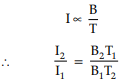Given, I1 = 8 Am–1
B1 = 0.6 T
T= 4 K
B2 = 0.2 T
T2 = 16 K
Putting the values,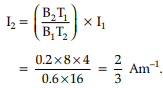Q.10. Can two equipotential surfaces intersect each other ? Justify your answer.  (1 Mark)
Ans: The normal drawn at any point on the equipotential surface will give the direction of electric field at that point. If the two surfaces intersect each other, there will be two directions of the electric field at that point which is not possible. So, the two equipotential surfaces cannot intersect each other.

For question numbers 11, 12, 13 and 14, two statements are given-one labelled Assertion (A) and the other labelled Reason (R). Select the correct answer to these questions from the codes (a), (b), (c) and (d) as given below:

Q.11. Assertion (A): When light wave travels from rarer to denser medium , its speed gets reduced and hence the energy also reduces.
Reason (R): Energy of wave is proportional to its velocity.  (1 Mark)
(a) Both A and R are true, and R is the correct explanation of A
(b) Both A and R are true, but R is NOT the correct explanation of A
(c) A is true, but R is false
(d) A is false, and R is also false
Ans: Correct option is (d)
Explanation: When light wave travels from rarer to denser medium, its speed and amplitude gets reduced and not the energy. So assertion is false.
Energy of wave is not proportional to its velocity. So reason is also false.

Q.12. Assertion (A): Compared to protons, the neutrons penetrate in the, matter more easily.
Reason (R): Mass of neutrons are slightly more than the mass of protons.  (1 Mark)
(a) Both A and R are true, and R is the correct explanation of A
(b) Both A and R are true, but R is NOT the correct explanation of A
(c) A is true, but R is false
(d) A is false, and R is also false
Ans: Correct option is (b)
Explanation: Protons being positively charged, they arerepelled by positively charged nucleus. But neutrons being neutral they are not repelled. Hence neutron has more penetrating power. Hence assertion is true.
Mass of proton = Mp=1.67265 a.m.u
Mass of neutron = Mn=1.67495 a.m.u
So assertion is also true. But R is not the proper explanation of A.

Q.13. Assertion(A): The energy (E) and momentum (p) of a photon is related as p = E/c (where c is the velocity of light ion vacuum)  (1 Mark)
Reason (R): The photon behaves like a particle.
(a) Both A and R are true, and R is the correct explanation of A
(b) Both A and R are true, but R is NOT the correct explanation of A
(c) A is true, but R is false
(d) A is false, and R is also false
Ans: Correct option is (a)
Explanation: For photon as a particle,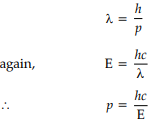Comparing two expressions of λ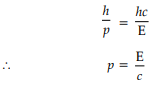So, assertion is true.
Through photoelectric effect experiment, Einstein showed that photon behaves like a particle. So, reason is also true. Reason also explains the assertion.

Q.14. Assertion (A): A red object appears dark in green light.
Reason (R): Red light scatters less.  (1 Mark)
(a) Both A and R are true, and R is the correct explanation of A
(b) Both A and R are true, but R is NOT the correct explanation of A
(c) A is true, but R is false
(d) A is false, and R is also false
Ans: Correct option is (b)
Explanation: A red object looks red because it reflects only red colour and absorbs all other colours . So the assertion is correct.
From Rayleigh's criterion scattering α 1/(wavelength)4
Red light has highest wavelength among the visible lights. So it scatters less. So, the reason is also true. But reason cannot explain the assertion.

Section B
Questions 15 and 16 are Case Study based questions and are compulsory. Attempt any 4 sub parts from each question. Each question carries 1 mark.
Q.15. Band theory of solid: Consider that the Si or Ge crystal contains N atoms. Electrons of each atom will have discrete energies in different orbits. The electron energy will be same if all the atoms are isolated, i.e., separated from each other by a large distance. However, in a crystal, the atoms are close to each other (2 Å to 3 Å) and therefore the electrons interact with each other and also with the neighbouring atomic cores. The overlap (or interaction) will be more felt by the electrons in the outermost orbit while the inner orbit or core electron energies may remain unaffected. Therefore, for understanding electron energies in Si or Ge crystal, we need to consider the changes in the energies of the electrons in the outermost orbit only. For Si, the outermost orbit is the third orbit (n = 3), while for Ge it is the fourth orbit (n = 4). The number of electrons in the outermost orbit is 4 (2s and 2p electrons). Hence, the total number of outer electrons in the crystal is 4N. The maximum possible number of outer electrons in the orbit is 8 (2s + 6p electrons). So, out of the 4N electrons, 2N electrons are in the 2N s-states (orbital quantum number l = 0) and 2N electrons are in the available 6N p-states. Obviously, some p-electron states are empty. This is the case of well separated or isolated atoms.

1. The energy of electrons of atoms of a substance will be same if:  (1 Mark)
(a) Atoms are isolated
(b) Atoms are closely spaced
(c) Atoms are excited
(d) Atoms are charged
Ans: Correct option is (a)
Explanation: The electron energy will be same if all the atomsare isolated, i.e., separated from each other by a large distance.

2. In a crystal, the distance between two atoms is:  (1 Mark)
(a) 200 Å to 300 Å
(b) 2 Å to 3 micron
(c) 2 Å to 3 Å
(d) 2 mm to 3 mm
Ans: Correct option is (c)
Explanation: In a crystal, the atoms are close to each other (2 to 3 Å).

3. The overlap (or interaction) will be more felt by the electrons when they are:  (1 Mark)
(a) in the outermost orbit
(b) in the innermost orbit
(c) free
(d) in any orbit
Ans: Correct option is (a)
Explanation: The overlap (or interaction) will be more felt by the electrons in the outermost orbit while the inner orbit or core electron energies may remain unaffected.

4. For Silicon and Germanium the outermost orbits are respectively  (1 Mark)
(a) n = 3 and n = 5
(b) n = 4 and n = 3
(c) n = 5 and n = 4
(d) n = 3 and n = 4
Ans: Correct option is (d)
Explanation: For Si, the outermost orbit is the third orbit (n = 3), while for Ge it is the fourth orbit (n = 4).

5. The maximum possible electrons in an orbit is  (1 Mark)
(a) 8 (2s + 6p electrons)
(b) 8 (6s + 2p electrons)
(c) 8 (4s + 4p electrons)
(d) 8 (1s + 7p electrons)
Ans: Correct option is (a)
Explanation: The maximum possible number of outer electrons in the orbit is 8 (2s + 6p electrons).

Q.16. Optical fibre works on the principle of total internal reflection. Light rays can be used to transmit a huge amount of data, but there is a problem here – the light rays travel in straight lines. So unless we have a long straight wire without any bends at all, harnessing this advantage will be very tedious. Instead, the optical cables are designed such that they bend all the light rays’ inwards (using TIR). Light rays travel continuously, bouncing off the optical fiber walls and transmitting end to end data. It is usually made of plastic or glass.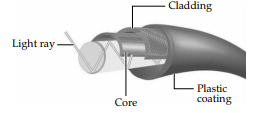Modes of transmission: Single-mode fiber is used for long-distance transmission, while multimode fiber is used for shorter distances. The outer cladding of these fibers needs better protection than metal wires. Although light signals do degrade over progressing distances due to absorption and scattering. Then, optical Regenerator system is necessary to boost the signal.
Types of Optical Fibers: The types of optical fibers depend on the refractive index, materials used, and mode of propagation of light. The classification based on the refractive index is as follows:
• Step Index Fibers: It consists of a core surrounded by the cladding, which has a single uniform index of refraction.
• Graded Index Fibers: The refractive index of the optical fiber decreases as the radial distance from the fiber axis increases.

1. Optical fiber works on the principle of:  (1 Mark)
(a) Scattering of light
(b) Diffraction of light
(c) Total internal reflection of light
(d) Dispersion of; light
Ans: Correct option is (c)
Explanation: The optical fiber works on the principle of total internal reflection.

2. For long-distance transmission  (1 Mark)
(a) Single mode fibre is used
(b) Multi mode fibre is used
(c) Both single mode and multimode are used
(d) Any one of single mode or multimode may be used.
Ans: Correct option is (a)
Explanation: Single-mode fiber is used for long-distance transmission, while multimode fiber is used for shorter distances.

3. Optical fibre is made of:  (1 Mark)
(a) Copper
(b) Semiconductor
(c) Plastic or glass
(d) Superconductors
Ans: Correct option is (c)
Explanation: Optical fiber is usually made of plastic or glass so that light rays can travel continuously, bouncing off the optical fiber walls and can be transmitting end to end data.

4. In graded index optical fibre:  (1 Mark)
(a) The refractive index of the optical fiber increases as the radial distance from the fiber axis increases
(b) The refractive index of the optical fiber decreases as the radial distance from the fiber axis increases
(c) The refractive index of the optical fiber remains same throughout
(d) Inner side of cladding is mirrored to ensure reflection
Ans: Correct option is (b)
Explanation: In graded Index Fibers, the refractive index of the optical fiber decreases as the radial distance from the fiber axis increases.

5. Light signal through optical fibre may degrade due to:  (1 Mark)
(a) Refraction
(b) Refraction and reflection
(c) Diffraction and scattering
(d) Scattering and absorption
Ans: Correct option is (d)
Explanation: Light signals do degrade over progressing distances due to absorption and scattering.

Section C
All questions are compulsory. In case of internal choices, attempt anyone.
Q.17. Draw a plot showing the variation of:
(i) electric field (E) and
(ii) electric potential (V)

with distance r due to a point charge Q.  (2 Mark)
Ans: Due to point charge
Electric field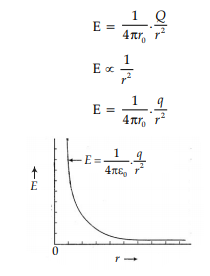Electric potential,V =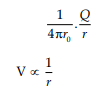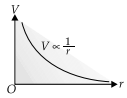Q.18. A 10 V cell of negligible internal resistance is connected in parallel across a battery of emf 200 V and internal resistance 38 W as shown in the figure.  (2 Mark)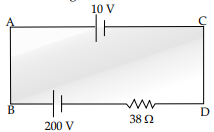Find the value of current in the circuit.
Ans: Writing the equation.
Finding the current.
Detailed Answer: By Kirchhoff's law, we have, for the loop ACDBA,
+ 200 – 38i – 10 = 0
i = 190/38 A = 5A
Alternatively: Finding the Net emf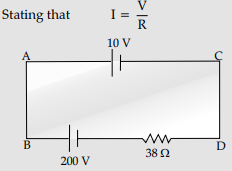Calculating I

The two cells being in 'opposition',

net E.M.F.    = 200−10 V=190 V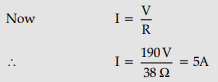[Note : Some students may use the formulae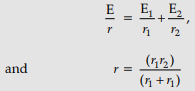For two cells connected in parallel, Then we can say that r = 0;
E is indeterminate and hence I is also indeterminate Award full marks (2) to students giving this line of reasoning.]

OR

Does the step down transformer violate the principle of conservation of energy ? Explain.  (2 Mark)
Ans: No, it does not violate the principle of conservation of energy. If a voltage is increased, the current is decreased in the same ratio and the product VI (power) remains constant.

Q.19. A parallel plate capacitor of capacitance C is charged to a potential V. It is then connected to another uncharged capacitor having the same capacitance. Find out the ratio of the energy stored in the combined system to that of stored initially in the single capacitor.  (2 Mark)
Ans: Energy stored in a capacitor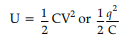Capacitance of the (parallel) combination = C + C = 2C
Here, the total charge, Q, remains the same :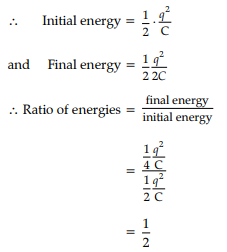OR

What is the relationship between the transformation ratio and the voltage?  (2 Mark)
Ans: If all the magnetic flux due to any current in the primary is linked with the secondary then,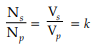where k is the transformation ratio.

Q.20. The figure shows two sinusoidal curves representing oscillating supply voltage and current in an ac circuit.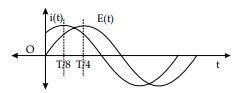Draw a phasor diagram to represent the current and supply voltage appropriately as phasors. State the phase difference between the two quantities.  (2 Mark)
Ans: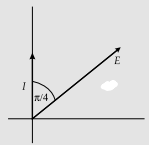Equal length of phasors,
Current leads voltage.
Phase difference is π/4
Detailed Answer:
The equation of voltage may be written as:
E(t) = E0 sin ωt    ...(I)
The equation of current may be written as :
I(t) = I0 sin (ωt + ϕ)  ...(ii)
where ϕ is the phase difference.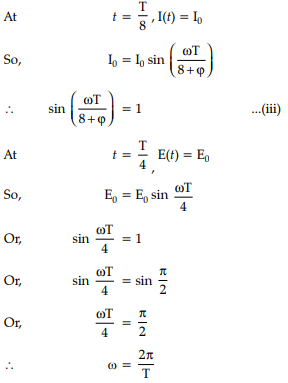Putting in equation (iii)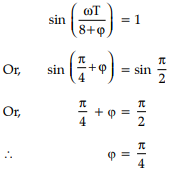So, phase difference = π/4
Current leads voltage by π/4
Phasor diagram: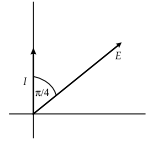Q.21. A small bulb (assumed to be a point source) is placed at the bottom of a tank containing water to a depth of 80 cm. Find out the area of the surface of water through which light from the bulb can emerge. Take the value of the refractive index of water to be 4/3.  (2 Mark)
Ans: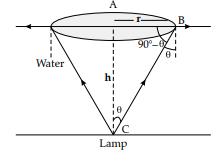Actual depth of the bulb in water, (h) = 0.8 m
Refractive index of water, μ = 4/3
where, Angle of incidence = q
Angle of refraction = 90°
Since the bulb is a point source, the emergent lightcan be considered as a circle of radius r.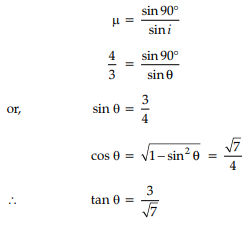From the figure, tan θ = r/h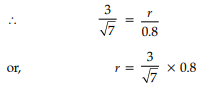∴  r = 0.91 m
Area of the surface of water through light emerge = πr2 = π (0.91)2 = 2.6 m2
Hence, the area of the surface of water through which the light from the bulb can emerge is approximately 2.6 m2

Q.22. An alternating voltage E = E0sin ωt is applied to the circuit containing a resistor R connected in series with a black box. The current in the circuit is found to be I = I0sin (ωt + π/4).
(i) State whether the element in the black box is a capacitor or inductor.
(ii) Draw the corresponding phasor diagram and find the impedance in terms of R.  (2 Mark)

Ans: (i) As the current leads the voltage by π/4, the element used in the black box is a ‘capacitor’.
(ii) Phasor diagram: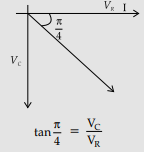VC = VR
XC = R
Impedance,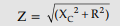Z = R √2

Q.23. A ray PQ incident normally on the refracting face BA is refracted in the prism BAC made of material of refractive index 1.5. Complete the path of ray through the prism. From which face will the ray emerge? Justify your answer.  (2 Mark)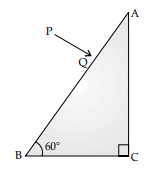Ans: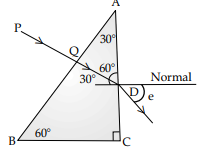At plane AC, the incident angle = 30°
If critical angle for total internal reflection = C
n = 1/sin C
or, sin C = 1/1.5 = 0.67 hence, C > 30° (sin 30° = 0.5)
As incident angle is less than critical angle, it would emerge out from AC.
In the figure, path of the ray is shown.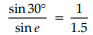or, sin e = 1.5 × sin 30°
= 1.5 × 0.5
= 0.75
∴ e = 48°.

Q.24. Compare the following :
(i) Wavelengths of the incident solar radiation absorbed by the earth’s surface
and the radiation re-radiated by the earth.
(ii) Tanning effect produced on the skin by UV radiation incident directly on the skin and that coming through glass window.  (2 Mark)

Ans: (i) Radiation radiated by earth has greater wavelength.
(ii) Tanning effect is significant for direct UV radiation; it is negligible for radiation coming through the glass.

OR

Write the expression for speed of electromagnetic waves in a medium of electrical permittivity E and magnetic permeability μ.  (2 Mark)
Ans: Formula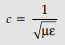Alternatively,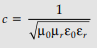Q.25. Identify the electromagnetic waves whose wavelengths vary as :
(i) 10–12 m < λ < 10–8 m
(ii) 10–3 m < λ < 10–1 m
Write one use for each.  (2 Mark)
Ans: (i) Identification
(ii) Uses
Detailed Answer: (i) X-rays
Used for medical purposes. (Also accept UV rays and gamma rays and anyone use of the EM wave named)
(ii) Microwaves
Used in radar systems. (Also accept short radio waves and anyone use of the EM wave named)

Section D

All questions are compulsory. In case of internal choices, attempt anyone.
Q.26. (i) Derive the expression for electric field at a point on the equatorial line of an electric dipole.
(ii) Depict the orientation of the dipole in (i) stable, (ii) unstable equilibrium in a uniform electric field.  (3 Mark)
Ans: Derivation of expression of electric field on equatorial line of electric dipole.
Depiction of orientation for stable and unstable equilibrium in electric field.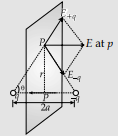(i) Let the point ‘P’ be at a distance ‘r’ from the mid point of the dipole.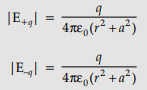Both are equal and their directions are as shown in the figure, hence net electric field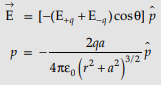(ii) Stable equilibrium q = 0°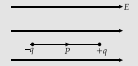Unstable equilibrium q = 180°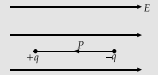Q.27. (i) Derive the expression for the electric potential due to an electric dipole at a point on its axial line.
(ii) Depict the equipotential surface due to electric dipole.  (3 Mark)
Ans: (i) Derivation of expression for electric potential due to electric dipole on axial line.
(ii) Depiction of equipotential surfaces due to an electric dipole
Detailed Answer: (i)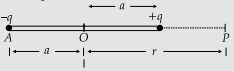Potential due to charge –q at A :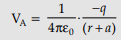Potential due to charge +q at B :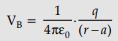Potential at point P, V = VA + VB
Net potential at point P :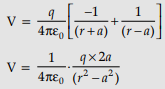(ii)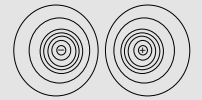OR

(a) In photoelectric effect, do all the electrons that absorb a photon come out as photoelectron irrespective of their location ? Explain.
(b) A source of light, of frequency greater than the threshold frequency, is placed at a distance ‘d’ from the cathode of a photocell. The stopping potential is found to be V. If the distance of the light source is reduced to d/n (where n > 1), explain the changes that are likely to be observed in the
(i) photoelectric current and (ii) stopping potential.  (3 Mark)
Ans: (a) No, it is not necessary that if the energy supplied to an electron is more than the work function, it will come out.
The electron after receiving energy, may lose energy to the metal due to collisions with the atoms of the metal. Therefore, most electrons get scattered into the metal.
Only a few electrons near the surface may come out of the surface of the metal for whom the incident energy is greater than the work function of the metal.
(b) On reducing the distance, intensity increases.
Photoelectric current increases with the increase in intensity.
Stopping potential is independent of intensity, and therefore remains unchanged.

Q.28. Using Kirchhoff's rules, calculate the potential difference between B and D in the circuit diagram as shown in the figure :  (3 Mark)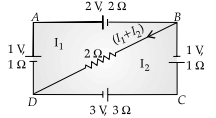Ans: Writing the two loop equations.
Finding the current through DB
Finding the p.d. between B and D
Detailed Answer: Using Kirchhoff's voltage rule, we have :
For loop DABD
(1 × I1 + (1) + (–2) + 2I1 + 2(I1 + I2) = 0
or 5I1 + 2I2 = 1 ...(i)
For loop DCBD
+ I2 × 3 + (3) + (–1) + 1 × I2 + 2(I1 + I2) = 0
or 2I1 + 6I2 = –2 ...(ii)
Solving (i) and (ii), we get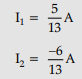∴   Current through DB = I1 + I2 =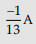∴   P.D. between B and D = 0.154 A

OR

An electron of mass me revolves around a nucleus of charge +Ze. Show that it behaves like a tiny magnetic dipole. Hence, prove that the magnetic moment associated with it is expressed as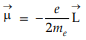where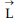is the orbital angular momentum of the electron.  (3 Mark)
Ans: (i) Behaviour of revolving electron as a tiny magnetic dipole.
(ii) Proof of the relation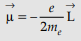(iii) Significance of negative sign.
Electron, in circular motion around the nucleus constitutes a current loop which behaves like a magnetic dipole.
Current associated with the revolving electron :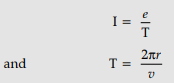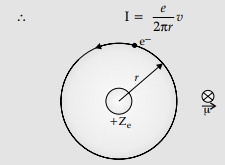Magnetic moment of the loop,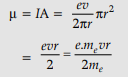Orbital angular momentum of the electron,
L = mevr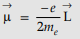–ve sign signifies that the angular momentum of the revolving electron is opposite in direction to the magnetic moment associated with it.

Q.29. State Lenz’s law. Explain, by giving examples that Lenz’s law is a consequence of the conservation of energy.  (3 Mark)
Ans: Statement of Lenz’s Law.
Explanation (with example).
Detailed Answer:
Statement: The polarity of induced emf is such that it tends to produce a current which opposes the change in magnetic flux that produced it.
Explanation: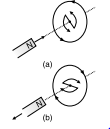When the north pole of a bar magnet is pushed towards the close coil, the magnetic flux through coil increases and the current is induced in the coil in such a direction that it opposes the increase in flux. This is possible when the induced current in the coil is in the anti clockwise direction. The opposite will happen when the north pole is moved away from the coil.
In either case, it is the work done against the force of magnetic repulsion/attraction that gets ‘converted‘ into the induced emf.

Q.30. Draw a circuit diagram of a potentiometer. State its working principle. Derive the necessary formula to describe how it is used to compare the emf's of the two cells.  (3 Mark)
Ans: The circuit diagram of the potentiometer, is as shown here: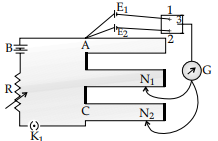Working Principle: The potential drop, V, across a length l of a uniform wire, is proportional to the length l of the wire.
or, V ∝ l (for a uniform wire)
Derivation: From the figure, connect points 1 and 3 together with balance point at point N1 where AN1 = l1 Now connect points 2 and 3 together with balance point at point N2 where AN2 = l2.
We have,
So, E1 = kl
and E2 = kl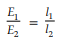Section E

All questions are compulsory. In case of internal choices, attempt anyone.
Q.31. (i) Describe the working principle of a step-up transformer with the help of a suitable diagram. Obtain the relation between input and output voltages in terms of the number of turns of primary and secondary windings and the currents in the input and output circuits
(ii) Given the input current 15 A and the input voltage of 100 V for a step-up transformer having 90% efficiency, find the output power and the voltage in the secondary if the output current is 3 A.  (5 Mark)

Ans: (i) Diagram
Principle
Relation between voltage, number of turns, and Currents
(ii) Input power
Output power
Output voltage
Detailed Answer: (i)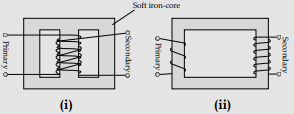Working principle: Whenever current in one coil changes, an emf gets induced in the neighbouring coil,
Voltage across secondary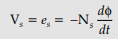Voltage across primary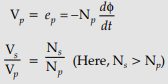In an ideal transformer

Power Input = Power output
IpVp = IsVs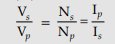(ii) Input power, Pi = Ii × Vi = 15 × 100 = 1500 W
Power output, P0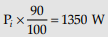⇒ I0V0 = 1350 W
Output voltage, V0 =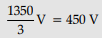OR

(i) Draw a ray diagram to show the image formation by a combination of two thin convex lenses in contact. Obtain the expression for the power of this combination in terms of the focal lengths of the lenses.
(ii) A ray of light passing from air through an equilateral glass prism undergoes minimum deviation when the angle of incidence is 3/4 th of the angle of prism. Calculate the speed of light in the prism.  (5 Mark)

Ans: (i)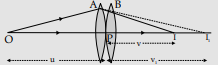Two thin lenses, of focal length f1 and f2 are kept in contact. Let O be the position of object and let u be the object distance. The distance of the image (which is at I1), for the first lens is v1.
This image serves as object for the second lens.
Let the final image be at I. We then have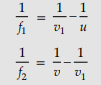Adding, we get

∴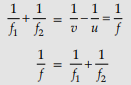∴ P = P1 + P
(ii) At minimum deviation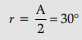We are given that,

∴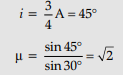∴ Speed of light in the prism = c/√2  (≡ 2.1 × 108 ms–1)
[Award ½ mark if the student writes the formula: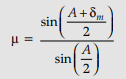but does not do any calculations.]

Q.32. (i) Explain the two features to distinguish between the interference pattern in Young’s double slit experiment with the diffraction pattern obtained due to single slit.
(ii) A monochromatic light of wavelength 500 nm is incident normally on a single slit of width 0.2 mm to produce a diffraction pattern. Find the angular width of the central maximum obtained on the screen.
Estimate the number of fringes obtained in Young’s double slit experiment with fringe width 0.5 mm, which can be accommodated within the region of total angular spread of the central maximum due to single slit.  (5 Mark)

Ans: (i) Differences:

 Interference Diffraction All maxima have equal intensity Maxima have different (rapidly decreasing) intensities All fringes have equal width Different (changing) width Superposition of two wavefronts Superposition of wavelets from the same wavefronts

(ii) Angular width of central maximum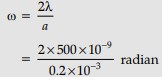= 5 × 10–3 radian
Now,  β = λD/d
Linear width of central maxima in the diffraction pattern
β' = 2λD/d
Let ‘n‘ be the number of interference fringes which can be accommodated in the central maxima.
n × β = β’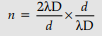n = 2

OR

(i) Describe the working principle of a step-up transformer with the help of a suitable diagram. Obtain the relation between input and output voltages in terms of the number of turns of primary and secondary windings and the currents in the input and output circuits.
(ii) Given the input current 15 A and the input voltage of 100 V for a step-up transformer having 90% efficiency, find the output power and the voltage in the secondary if the output current is 3 A.  (5 Mark)

Ans: (i) Diagram.
Principle.
Relation between voltage, number of turns, and Currents.
(ii) Input power.
Output power.
Output voltage.
Detailed Answer: (i)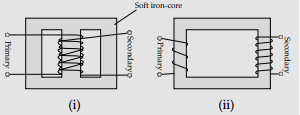Working principle: Whenever current in one coil changes, an emf gets induced in the neighbouring coil,
Voltage across secondary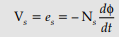Voltage across primary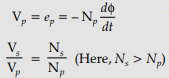In an ideal transformer
Power Input = Power output
IpVp = IsVs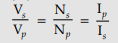(ii) Input power, Pi = Ii × Vi = 15 × 100 = 1500 W
Power output, P0 =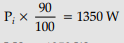⇒ I0V0 = 1350 W
Output voltage, V0 =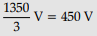Q.33. (i)Define electric flux. Is it a scalar or a vector quantity ? A point charge q is at a distance of d/2 directly above the centre of a square of side d, as shown in the figure. Use Gauss's law to obtain the expression for the electric flux through the square.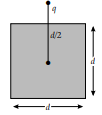(ii) If the point charge is now moved to a distance ‘d’ from the centre of the square and the side of the square is doubled, explain how the electric flux will be affected.  (5 Mark)
Ans: (i) Definition of electric flux.
Stating scalar/ vector.
Gauss's law.
Derivation of the expression for electric flux.
(ii) Explanation of change in electric flux.
Detailed Answer: (i) Electric flux through a given surface is defined as the dot product of electric field and area vector over that surface.
Alternatively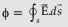Electric flux, through a surface equals the surface integral of the electric field over that surface.
It is a scalar quantity.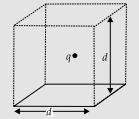Constructing a cube of side 'd' so that charge 'q' gets placed within of this cube (Gaussian surface )
According to Gauss's law the Electric flux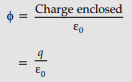This is the total flux through all the six faces of the cube.
Hence electric flux through the square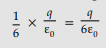(ii) If the charge is moved to a distance d and the side of the square is doubled the cube will be constructed to have a side 2d but the total charge enclosed in it will remain the same. Hence the total flux through the cube and therefore the flux through the square will remain the same as before.
[Deduct 1 mark if the student just writes No change/not affected without giving any explanation.]

OR

(a) Explain with the help of suitable diagram, the two processes which occur during the formations of a p-n junction diode. hence define the terms:
(i) depletion region and
(ii) potential barrier.
(b) Draw a circuit diagram of a p-n junction diode under forward bias and explain its working.  (5 Mark)

Ans: (a) Explaining the two processes.
Defining the two terms.
(b) Circuit diagram.
Working.
Detailed Answer: (a) The two important processes are diffusion and drift.
Due to concentration gradient, the electrons diffuse from the n side to the p side and holes diffuse the p side to the n side.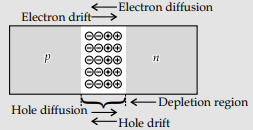Due to the diffusion, an electric field develops across the junction. Due to the field, an electron moves from the p-side. The flow of the charge carriers due to the electric field, is called drift.
(i) Depletion region: It is the space charge region on either side of the junction, that gets depleted of free charges is known as the depletion region.
(ii) Potential Barrier: The potential difference, that gets developed across the junction and opposes the diffusion of charge carries and brings about a condition of equilibrium, is known as the barrier potential.
(b)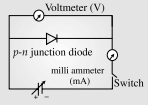In forward bias, p-side of the diode is connected to the positive terminal of the battery and N-side is connected to negative terminal of the battery.
In forward bias, applied voltage does not support potential barrier. As a result, the depletion layer width decreases and barrier height is reduced. Due to the applied voltage, electrons from n side cross the depletion region and reach p side. Similarly holes from p side cross the junction and reach the n side.

Offer running on EduRev: Apply code STAYHOME200 to get INR 200 off on our premium plan EduRev Infinity!

67 docs

,

,

,

,

,

,

,

,

,

,

,

,

,

,

,

,

,

,

,

,

,

;